Electron. J. Differential Equations, Vol. 2019 (2019), No. 41, pp. 1-23.

### Multiplicity and concentration of nontrivial solutions for generalized extensible beam equations in R^N Juntao Sun, Tsung-Fang Wu

Abstract:
In this article, we study a class of generalized extensible beam equations with a superlinear nonlinearity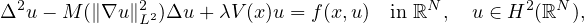where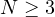,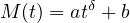with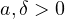and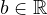,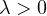is a parameter,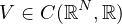and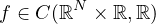. Unlike most other papers on this problem, we allow the constant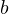to be non-positive, which has the physical significance. Under some suitable assumptions on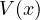and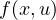, when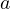is small and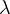is large enough, we prove the existence of two nontrivial solutions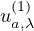and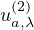, one of which will blow up as the nonlocal term vanishes. Moreover,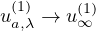and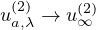strongly in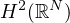as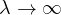, where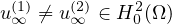are two nontrivial solutions of Dirichlet BVPs on the bounded domain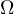. Also, the nonexistence of nontrivial solutions is also obtained forlarge enough.

Submitted December 1, 2018. Published March 19, 2019.
Math Subject Classifications: 35J30, 35J35.
Key Words: Extensible beam equations; nontrivial solution; multiplicity; concentration of solutions.

Show me the PDF file (422 KB), TEX file for this article.Juntao Sun School of Mathematics and Statistics Shandong University of Technology Zibo 255049, China email: jtsun@sdut.edu.cn Tsung-Fang Wu Department of Applied Mathematics National University of Kaohsiung Kaohsiung 811, Taiwan email: tfwu@go.nuk.edu.tw